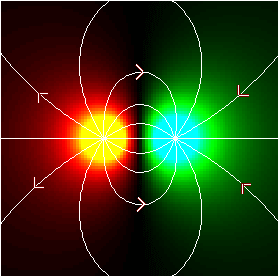### The Electric Field

1. The electric field is conservative, so
F = - ∇ U = - qt ∇ V.
We define the (vector) electric field (Coulomb's law):
E(x,y,z) ≡ - ∇ V(x,y,z)
= (1 / (4 π ε)) ∫ r dq / r3
The vector r in the integrand is the vector pointing from the charge dq to the field point. The integrand is most often written as q times the unit vector pointing in the r direction, divided by r2, but this expression is more useful unless the problem is 1-dimensional. Either way, the field (and therefore the force) decreases as 1/r2.

Note that the electric field has units both of N / C and V / m. Think of it both as a force per unit charge and a potential per unit distance.

The direction of the electric field at a point is defined as the direction of the acceleration experienced by a positive test charge:Since the electric field is minus the gradient of the potential field,

ΔV = - ∫ E ⋅ ds,
where the integral is along a path between two field points.

Consider again the line of charge extending from -b to b. We could compute the electric field using

E(x,y) = (λ / (4 π ε)) ∫-bb (x - s, y) ds / (√ ((x - s)2 + y2))3
or we can use
E(x,y) = - ∇ V(x,y)
= (λ / (4 π ε)) (1 / √((b - x)2 + y2) - 1 / √((b + x)2 + y2),
((b - x) / y) / √((b - x)2 + y2) + ((b + x) / y) / √((b + x)2 + y2))
If the field point is on the y axis, this becomes
E(y) = λ b / (2 π ε y √ (b2 + y2)) j
In the limit b → ∞, this is
E(x,y) = λ / (2 π ε y) j
2. Always check to see if one or more components of the electric field are zero because of symmetry of the source charge configuration.
3. When the charges are in equilibrium, the electric field is zero inside a conductor, and normal to its surface.

 (prev) (top) (next)